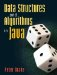# Timing

If we want to know which of two methods is faster, the most obvious approach is to time them. The TimeTest program (Figure 7-1) compares the get() methods from the ArrayList and LinkedList classes in the Java collections framework.

Lines 1416 of the method test() add many elements to list. We don't care what these elements are, so this is one of those rare occasions where it makes sense to create instances of the Object class.

To determine the time taken by line 18, we examine the clock before and after invoking get(). To get the current time, we invoke the static currentTimeMillis() method of the System class. For historical reasons involving the UNIX operating system, this is given as the number of milliseconds since midnight, January 1, 1970, Greenwich mean time. Because this is a very large number, the return type of currentTimeMillis() is long. A long is similar to an int, but its range is roughly ±1019.

Figure 7-1. The TimeTest class compares the get() methods of the ArrayList and LinkedList classes.

 ``` 1 import java.util.*; 2 3 /** Time tests to compare performance of various algorithms. */ 4 public class TimeTest { 5 6 /** Number of Objects to store in each data structure. */ 7 public static final int LIST_LENGTH = 100; 8 9 /** 10 * Store LIST_LENGTH Objects in list. Time list's get() method, 11 * printing the number of milliseconds taken. 12 */ 13 public static void test(List list) { 14 for (int i = 0; i < LIST_LENGTH; i++) { 15 list.add(new Object()); 16 } 17 long before = System.currentTimeMillis(); 18 list.get(5); 19 long after = System.currentTimeMillis(); 20 System.out.println((after - before) + " milliseconds"); 21 } 22 23 /** Compare ArrayList with LinkedList. */ 24 public static void main(String[] args) { 25 System.out.print("ArrayList: "); 26 test(new ArrayList()); 27 System.out.print("LinkedList: "); 28 test(new LinkedList()); 29 } 30 31 }```

Unfortunately, running our program doesn't give us much information:

```ArrayList: 0 milliseconds
```

Any modern computer is so fast that, on either of these data structures, the get() method takes less than one millisecond to run. A reasonable solution is to look at the time required to perform each operation a million times (Figure 7-2).

Figure 7-2. We can improve the resolution of our timing by performing the operation in question many times.

 ``` 1 /** Number of times to perform the operation being timed. */ 2 public static final int TEST_RUNS = 1000000; 3 4 /** 5 * Store LIST_LENGTH Objects in list. Time list's get() method, 6 * printing the number of milliseconds taken. 7 */ 8 public static void test(List list) { 9 for (int i = 0; i < LIST_LENGTH; i++) { 10 list.add(new Object()); 11 } 12 long before = System.currentTimeMillis(); 13 for (int i = 0; i < TEST_RUNS; i++) { 14 list.get(5); 15 } 16 long after = System.currentTimeMillis(); 17 System.out.println((after - before) + " milliseconds"); 18 }```

The exact result of running this program varies from one run to the next. For example, one run might give

```ArrayList: 21 milliseconds
```

while another might give

```ArrayList: 22 milliseconds
```

This variation is due to a number of factors beyond our control, such as how Java manages its memory (Chapter 16) and what other programs are running on our machine. We could try harder to control these conditions and apply various statistical techniques to determine which method is faster, but the short answer is that the method from ArrayList is roughly twice as fast as the method from LinkedList. They are both very fast, so this is not a big difference.

The story is different if we get element 50 instead of element 5. Now the method from LinkedList takes vastly longer:

```ArrayList: 22 milliseconds
```

In retrospect, this is not surprising. In an ArrayList, get() jumps right to the array element in question. In a LinkedList, it is necessary to traverse all of the previous list nodes to find the one we want. Our timing experiment provides empirical evidence that the time to get element i of a LinkedList increases with i, while the time to get element i of an ArrayList is independent of i. If we need a List and get() is going to be a very common operation, an ArrayList is a good choice.

We now have some evidence that LinkedList search time increases with i, but a couple of data points are not really a convincing case. Rather than perform a more extensive battery of tests, we can use some formal, mathematical tools to make statements about the efficiency of an algorithm. Section 7.2 introduces these tools.

The material that follows is important for designing general-purpose algorithms and data structures. Empirical timing is still important in optimization.

Exercises

 7.1 The famous Y2K bug occurred because some old programs used two decimal digits to store years. This became a problem in the year 2000, because such programs had no way to tell whether "00" meant 1900 or 2000. A similar problem will occur for Java programs when the number of milliseconds since the beginning of 1970 exceeds the capacity of a long. In what year will this occur, given that the maximum value of a long is 9,223,372,036,854,775,807? What if getTime() returned an int, which has a maximum value of 2,147,483,647? What about those UNIX/C systems which use an int to store the number of seconds since the beginning of 1970?Data Structures and Algorithms in Java
ISBN: 0131469142
EAN: 2147483647
Year: 2004
Pages: 216
Authors: Peter Drake